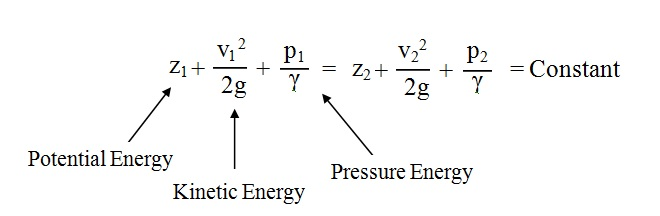# Mechanical Engineering

Basics of Mechanical Engineering

## LATEST

For Ideal fluid:

The Bernoulli's equation states that, For a perfect incompressible liquid, flowing in a continuous stream, the total energy of a particle remains the same, while the particle moves from one point to another.For Real fluid:

The Bernoulli's equation for real fluids is given byWhere,
hL = Loss of energy between sections 1 and 2